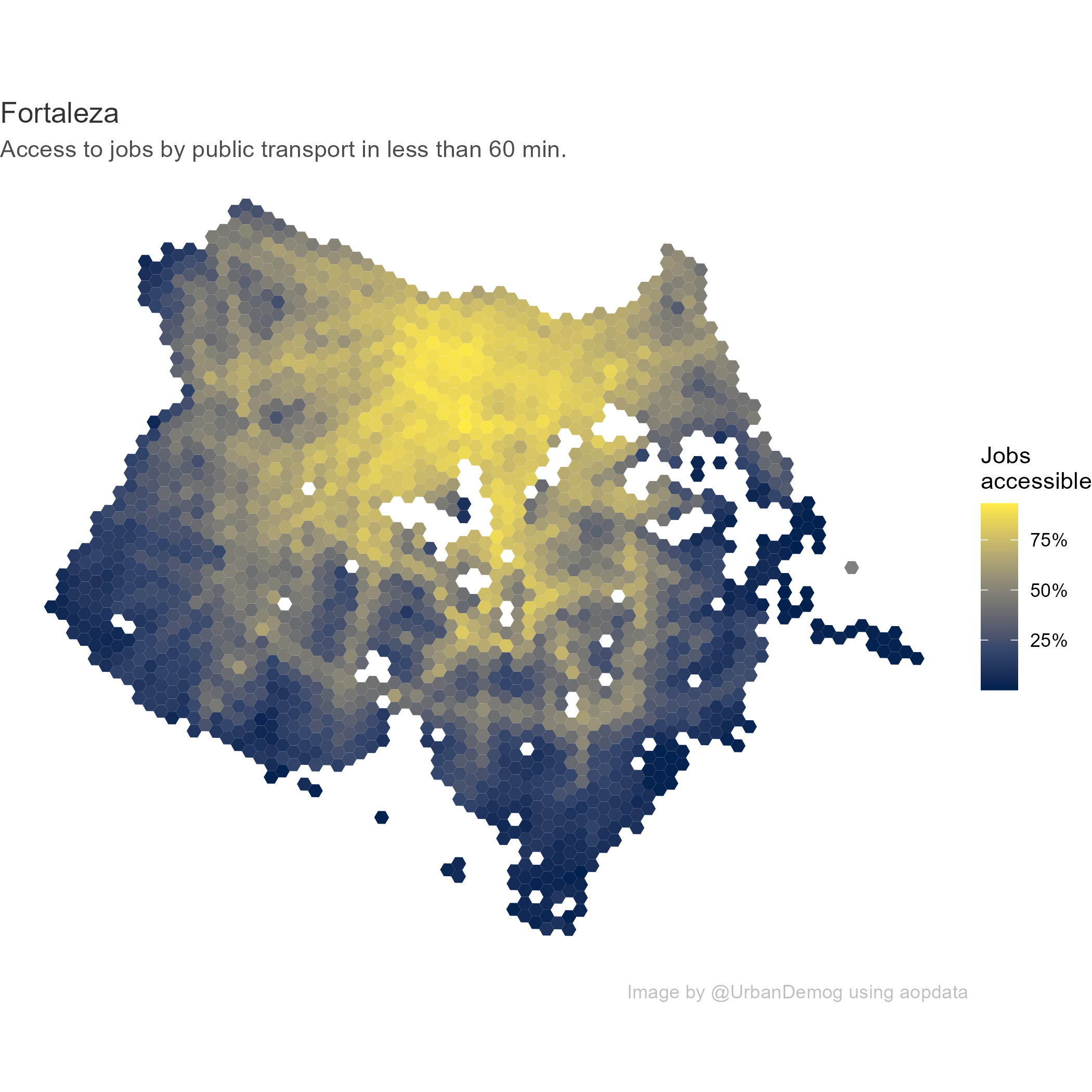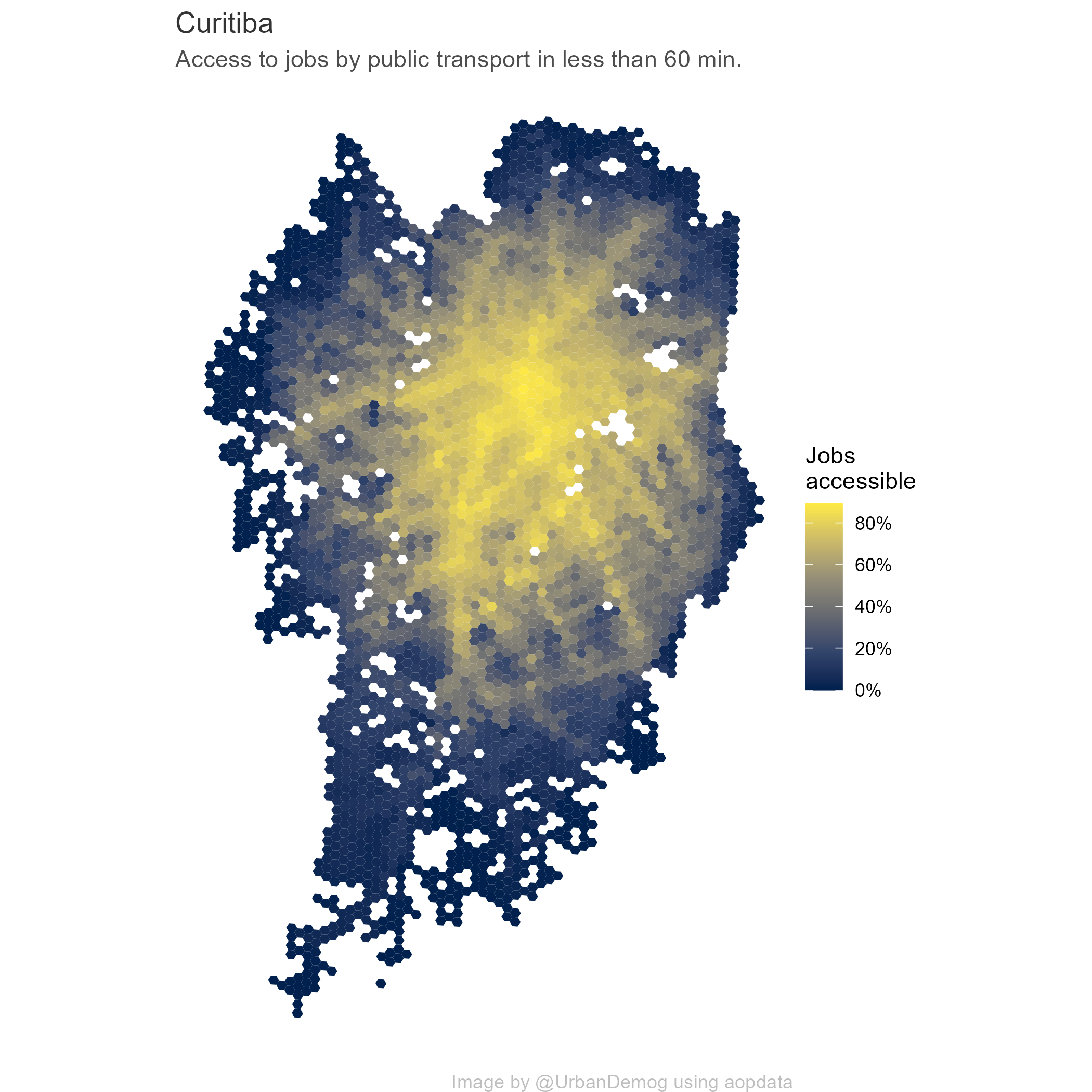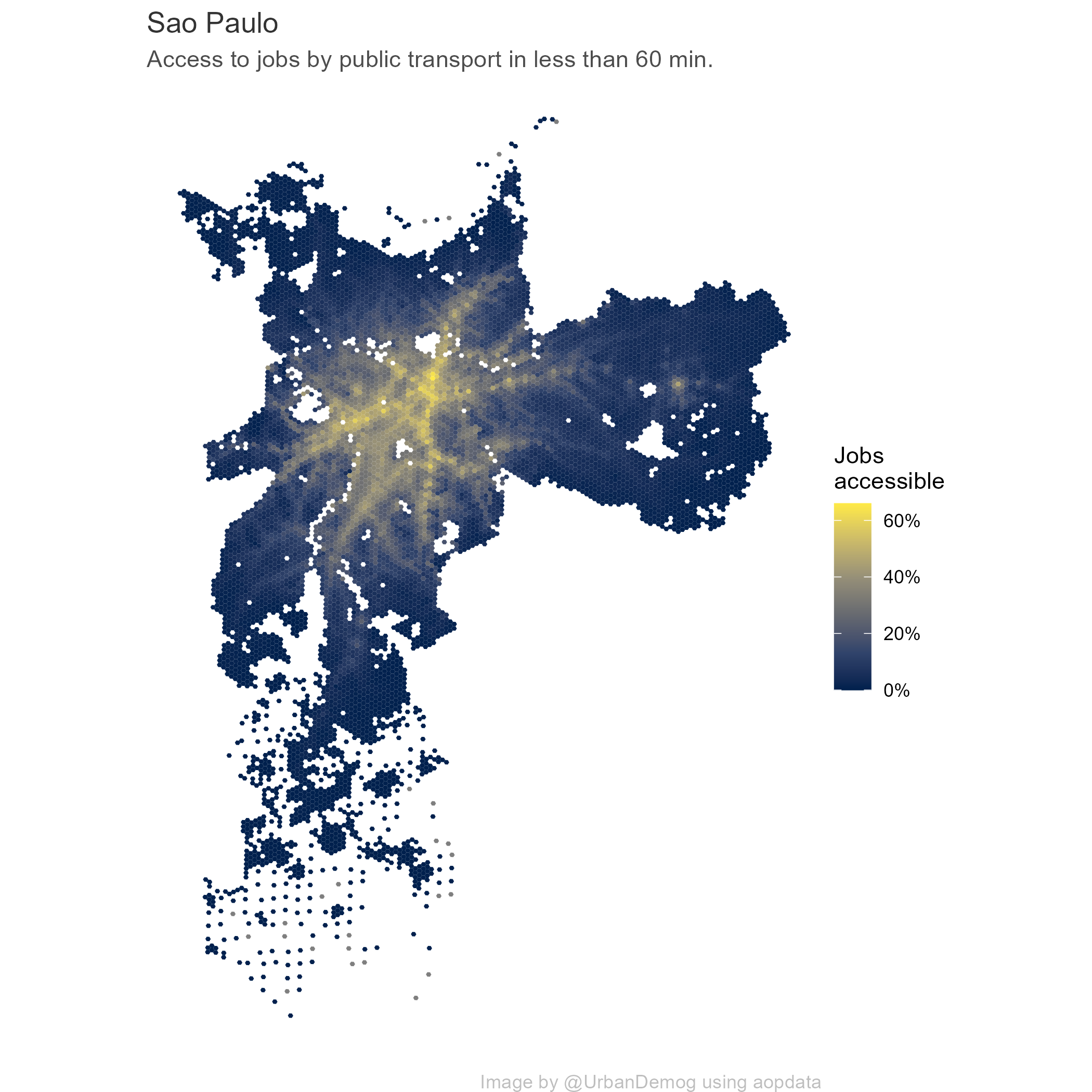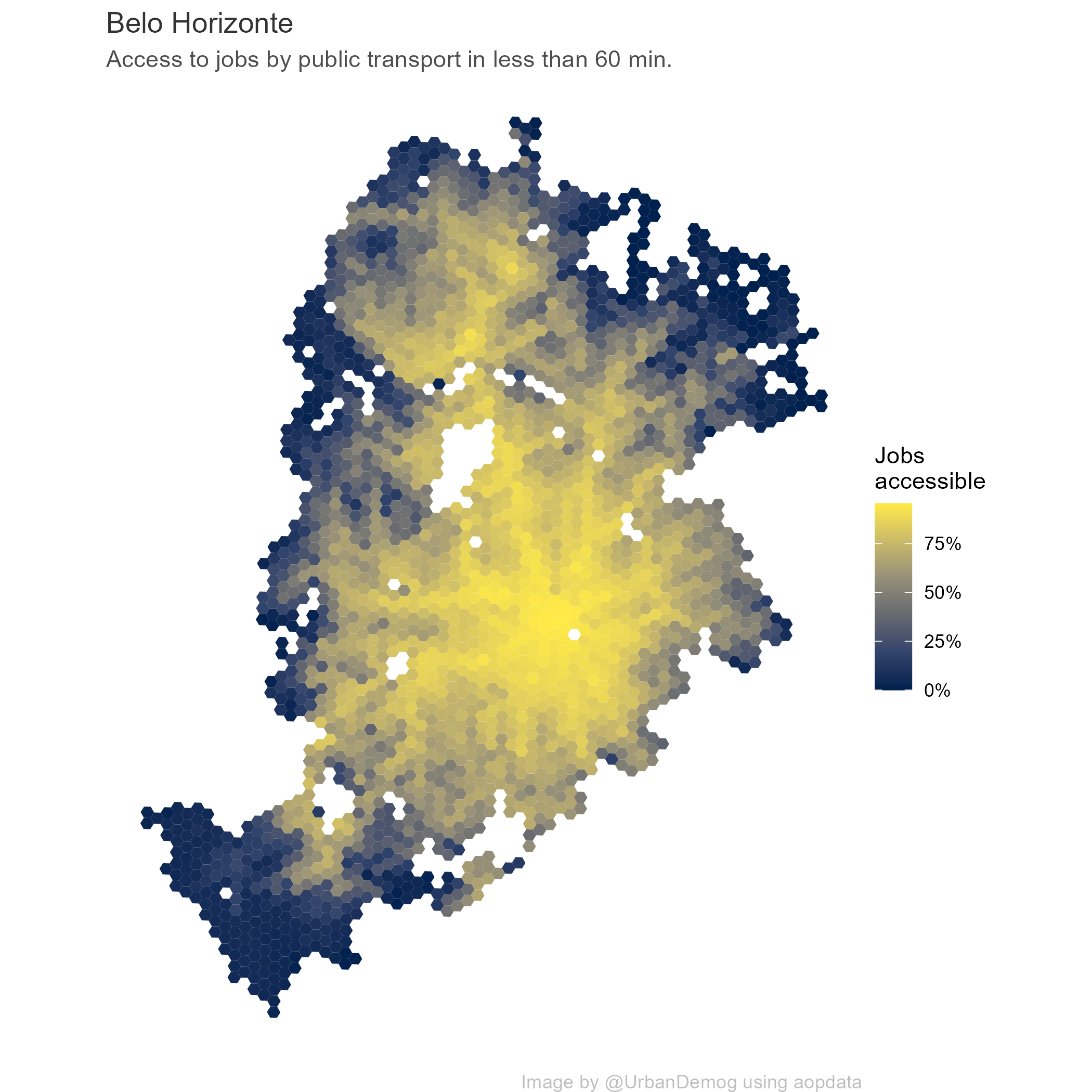The topic of day 4 in the #30DayMapChallenge is ‘Hexagons’. I thought this could be a good opportunity to show how one can download and map the data we generate in the Access to Opportunities Project.

So here is a quick code snippet to map employment accessibility by public transport in some of Brazil’s cities. The data can be easily downloaded from the aopdata package.

``````
library(aopdata)
library(ggplot2)

cities_list <- c('Fortaleza', 'Curitiba', 'Sao Paulo', 'Porto Alegre')
a <- read_access(city=cities_list, mode = 'public_transport', geometry = T)

# function to generate plots
save_plot <- function(i){ # i="Sao Paulo"

# select city
temp_df <- subset(a, name_muni== i)
temp_df <- subset(temp_df, P001 > 0)

temp_fig <- ggplot() +
geom_sf(data=temp_df, aes(fill=CMATT60), color=NA) +
scale_fill_viridis_c(option = "cividis", labels=scales::percent) +
title=paste0(i) ,
fill="Jobs \naccessible",
caption = 'Image by @UrbanDemog using aopdata') +
theme_void() +
theme(plot.title = element_text(color = "gray20"),
plot.subtitle = element_text(color = "gray30"),
plot.caption = element_text(color = "gray")
)
# save
ggsave(temp_fig, filename = paste0(i,'.png'), width = 7, height = 7, dpi=300)

}

# run :)
lapply(X=cities_list, FUN=save_plot)

``````